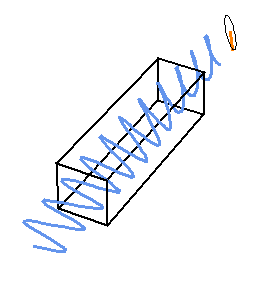### Optical Activity and Light Polarization

Chiral molecules are molecules that do not have a plane of symmetry, an inversion center, or so-called improper rotation symmetry axes. For example, the water molecule has a plane of symmetry but sugar molecules don’t. See this page for an illustration of various types of molecular chirality. As a consequence, chiral molecules such as sugars, amino acids, and most other bio-molecules, come in two forms (enantiomers) that are mirror images of each other and that are not the same. The concept of chirality is not restricted to molecules; one can also speak of chiral objects in general terms. The term ‘chiral’ means ‘handedness’ and refers to the fact that our hands are mirror images of each other [when you press a hand flat against a mirror it looks the same as if you press your hands flat together] but a hand and its mirror image are not identical and you cannot superimpose them. Chiral objects always come in pairs, conceptually. Back to molecules: Two enantiomers have the same chemical reactivity, the same mass, volume, and so on. One can distinguish them most easily in two ways: First, enantiomers react differently with other chiral molecules. Second, chiral molecules are said to be optically active.

#### Optical rotation

Two phenomena play an important role in the context of natural optical activity, that is to say, optical activity that occurs without applying magnetic fields and such. One of them is optical rotation. Optical rotation means the the plane of polarization of a linearly polarized light beam rotates as it passes through an optically active medium such as a solution of chiral molecules. The rotation angle is proportional to the path length through the medium, and in case of a solution it is also proportional to the concentration of the chiral substance.

The graphics below shows an animation of a linear polarized light wave as it passes through an optically active medium (indicated by the rectangular box). The plane of polarization of the light wave rotates proportional to the path length. The box dimension, the amount of rotation, and the amplitudes are not to scale. Optical rotation is measured by polarimetry. The wavelength used in polarimetry experiments is often 589.3 nm (this is the yellow light from a sodium lamp). Optical rotation data are typically given in terms of a path length of 10 cm (1/3 foot).Identically prepared solutions of the two enantiomers of a chiral molecule rotate the polarization plane in equal but opposite directions. A mixture of equal amounts of the two enantiomers (racemate) or a nonchiral substance does not exhibit this effect. However, the sign of the rotation is not in a simple way related to the chiral structure in the sense that, say, a D configuration would always have a positive or negative optical rotation. Therefore, without additional structural information it is not possible to deduce an unknown absolute configuration of a molecule simply from the sign of the optical rotation. Quantum theoretical calculations are very useful in this case.

#### Circular Dichroism

Another important phenomenon that is only observed for chiral substances is called circular dichroism (CD). In a nutshell, if we record the electronic absorption spectrum (typically in the UV-Vis range) of a chiral substance with circularly polarized light (see explanations below) instead of linearly polarized light, the spectra for one of the enantiomers recorded with left or right circularly polarized light differ slightly. Compare the red and green curves in the image below. (See the next paragraph for some animations of circularly and elliptically polarized waves and further explanations). If we plot the difference between the two absorption spectra we have what is called the CD spectrum shown in the graphics below in blue (here: ‘red minus green’). The CD spectrum (the Δ𝜀 curve) for the other enantiomer would have the opposite sign, i.e. we would obtain the blue curve mirrored at the horizontal axis. Nonchiral substances do not exhibit CD except in the presence of a static magnetic field (Faraday effect).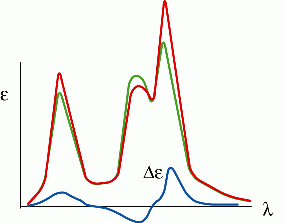#### Circularly Polarized Light

The fact that the absorption spectra of a chiral substance measured with left and right circularly polarized light differ somewhat can be rationalized by the fact that circularly polarized light is ‘chiral’ in itself. Why is that? Helical objects are chiral. Look at the pictures below (in case you are curious, this is a bike stand; I forgot where I took the photo):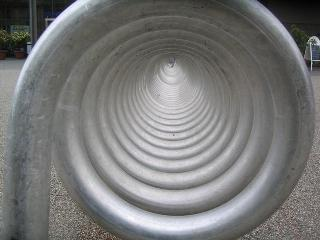Any helical object like this one has a mirror image that is also helical but with the opposite sense of rotation. The mirror image and the original are not identical. In a sense, every chiral molecule also has such a helicity built into it.

The two forms of circular polarization of light (left or right) are mirror images of each other. First, let’s see what a circularly polarized light wave looks like. We consider only the electric field component of the electromagnetic wave. Suppose you superimpose (add) two linearly polarized electromagnetic waves with the same amplitude and frequency (wavelength) but where the electric field vector in the one case oscillates, say, in the xz plane and in the other case it oscillates in the yz plane. Suppose the waves propagate in the z direction. If the two waves have a phase shift of exactly one quarter of the wavelength, voila! We obtain a light wave where the resulting electric field vector at any point along the z direction turns around on a circle either clock-wise or anti-clock-wise. This is why we call this light wave circularly polarized. See the animation below on the left. In the case that the two amplitudes are not exactly equal, the electric field vector rotates clock-wise or anti-clock-wise on an ellipse instead and we obtain elliptically polarized light. See the animation below on the right. You can also download interactive versions of these animations by following the Download links. You need the Wolfram Player (free download from the Wolfram research web site)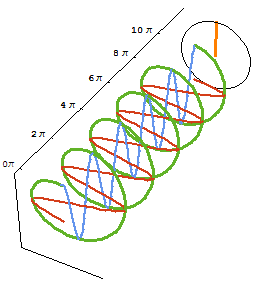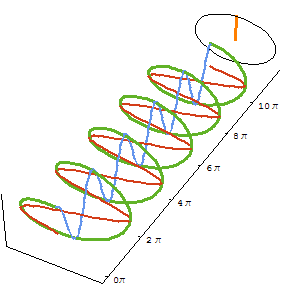Circular Polarization Elliptic Polarization and change the amplitude ratio or phase shift to switch between circular and elliptic polarization

In both animations the electric field vector (orange) at a fixed position z in space (here at 12 π) is shown in addition to the wave itself as it propagates. The circular and elliptic polarizations are clearly visible. In blue and red we have the two individual linearly polarized light beams and in green their superposition. Because the ‘green wave’ has the shape of a helix it can be considered chiral in some sense, because there is a left-handed and a right-handed helix which are mirror images of each other. In the animations above, the blue wave is one quarter of a wavelength ahead of the red one. If it were behind instead, we would obtain circularly or elliptically polarized light with the opposite helicity.

These two forms of circularly polarized light interact slightly differently with a chiral molecule, which causes the circular dichroism. A different interaction of the two forms of circularly polarized light with an enantiomer of a chiral molecule also causes the optical rotation, which is explained further below.

As an example, consider the molecule ‘hexahelicene’. Its chemical formula is shown below on the left (‘hexa’ because of the 6 aromatic rings). The molecule adopts a helical shape as shown in the middle, otherwise the atoms at the ends of the molecule would get too close. There are two possible forms: a left-handed helical form as shown below (M for the ‘Minus’ sense of hecility), and its the mirror image which has a right-handed helical shape (not shown. This molecule is named (P)-hexahelicene, ‘Plus’). Because of its helical shape, the molecule is chiral. It has a very intense CD spectrum shown below on the right. In green is the experiment. The red spectrum is the result of a computation that we performed in 2002 (Ref. ) which agrees quite well with the experiment except at the shortest wavelengths where we cut off the computation of additional excitations. (P)-Hexahelicene would have a CD spectrum of the opposite sign.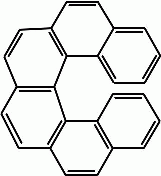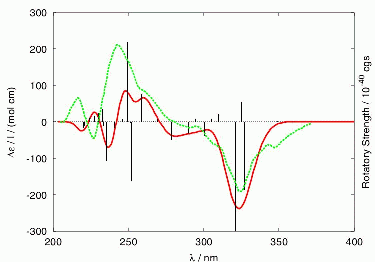hexahelicene (M)-hexahelicene CD spectrum of (M)-hexahelicene Green: Experiment Red: Computation

#### What causes the optical rotation?

Interestingly, a monochromatic linearly polarized light beam can be considered as a superposition of two circularly polarized electromagnetic waves that are propagating in the same direction with the same frequency but the opposite sense of rotation. Consider the animation of circularly polarized light above. If we superimpose this wave with a circularly polarized wave of the opposite ‘handedness’ where the blue component is 1/4 wavelength behind (instead of ahead), the two blue components will completely cancel because they are 180 degrees (half a wavelength) out of phase. Thus, we would be left with just a linearly polarized wave.

The plane of polarization of the resulting linearly polarized wave thus prepared can be changed (rotated) by applying a phase shift between its two circularly polarized components. With the help of this concept we can explain the phenomenon of optical rotation: We have seen that chiral molecules interact slightly differently with the two circularly polarized components of a linearly polarized light beam. This is true both for absorption and refraction. Left and right circularly polarized light beams also have slightly different refractive indices in a chiral medium. This means that left and right circularly polarized light travel waves at slightly different speeds through the medium. Therefore, this causes a phase shift between the two circularly polarized components, which increases proportionally to the path length that the light travels through the chiral medium. This phase shift manifests itself as a rotation of the plane of polarization of the resultant linearly polarized light beam—optical rotation. In fact this is exactly how the animation of optical rotation at the top of the page was created with the Mathematica software.

#### Ellipticity

Suppose we investigate optical rotation at a wavelength where the medium absorbs some of the light’s intensity. Because a chiral medium absorbs left and right circularly polarized light differently (the CD effect), the amplitudes of the outgoing two circularly polarized components of the light beam are not equal anymore after they have passed through the absorbing chiral medium. They have a phase shift and a different amplitude. We end up with a situation similar to the one depicted in the animation above of elliptic polarization (caused by the different amplitudes of the linearly polarized components). In other words, the outgoing light beam is not linearly polarized anymore. Instead, it is elliptically polarized. See the animation below. It was created with Mathematica in the same way as the animation at the top of the page, but with one of the circular components being reduced in amplitude as it passes through the optically active medium. The ellipticity of the outgoing light is clearly visible in the electric field vector (orange) at some fixed position.